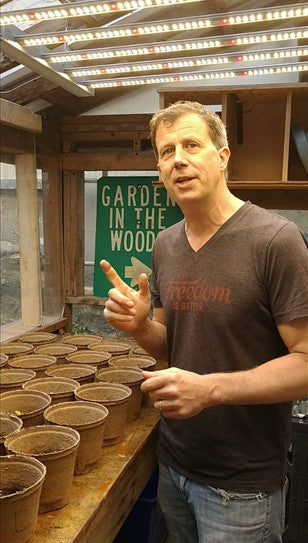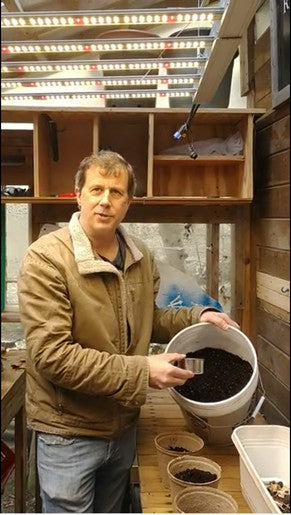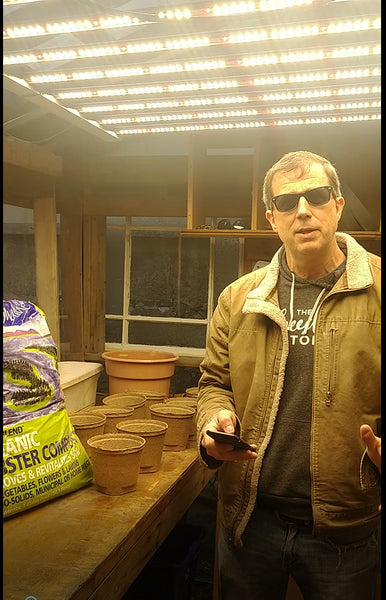# Understanding Watts, Amps and Volts

November 22, 2017

Understanding Watts, Amps and Volts

Every once and a while the question will arise, “is 240 Volts more efficient than running 120 Volts?”

In this entry we’ll explain that while 240V might be slightly more efficient, the electricity savings would be nominal.

When you receive your electric bill, what you are paying for is wattage used by your electrical devices, including your grow light fixtures. Volts and amps will not be a factor when looking at your electric bill and when you’re looking for electrical cost savings.

The number of watts an electrical device uses should be the main factor to look at when trying to scale back on your electrical costs, which is why many indoor growers are now turning to LED grow lights. To help explain why this is, lets first look at what watts, amps and volts are.

What are Volts, Amps, and Watts?

• Voltage (v)is the measure of electric pressure, or in other words, how hard electricity is sent through a wire. A good analogy to use would be water flow through a hose. In this example volts would represent your water pressure.
• Amperage (a)is the measure of the amount of electricity sent through a wire or circuit, also known as electric current. Using the same water flow analogy, amps are similar to the amount of water flowing through a hose in a certain amount of time.
• Wattage (w) is the amount of power that is required to operate an electrical device. To round out our water flow analogy, watts would be the power the water could provide (think back to when water was used to power mills). Wattage is the result of multiplying amps and volts together (amps x volts = watts), which is the working capacity of the electricity.

Electricity is the flow (like water) of electrons through a conductor like a wire. The rate at which electricity flows is measured as an electric current. The electric current is measured in amps. What makes the current flow? In our water analogy we could say a battery would be the pump that makes the water flow which creates pressure in the pipe. The pressure is the voltage. And as we said before the watts are the power the water could provide (like to a mill wheel). The watt is a measure of how much power is released each second. Your power company bills you based on the watts you use, not the voltage or amperage.

How Electrical Billing Works

Using 240V to run your electrical devices as a way to save on electrical costs is a common misunderstanding about how electricity works and how the power companies charge you for it. The point often noted for the money saving argument is that the amperage is half as much when running grow lights on 240 volts instead of 120 volts. This is true but the utility company doesn't charge you for amperage, they charge you for wattage. They bill you in kilowatt-hour units. A kilowatt-hour is 1000 watts of usage for one hour or approximately equals a 1000-watt light running for one hour. The formula for this is: Wattage / Voltage = Amperage. If we plug in the numbers for a 1000-watt high pressure sodium grow light, you can see that although the voltage and amperage can change, the wattage (what you are charged for) will always stay the same.

1000W / 240V = approx. 4A

1000W / 120V = approx. 8A

So, while using a higher voltage to run a 1000W HPS light reduces amperage, the wattage required to run the fixture remains the same and your electric bill will still reflect kilowatt-hours. The only way to noticeably reduce costs on your electrical bill would be reduce watts used.

### In Conclusion

When using electrical appliances or devices you are using watts. Wattage is how electrical utilities measure your electrical usage (in kilowatt/hr) and is ultimately what you are billed for, regardless of the amperage or voltage. If you are looking to reduce your electric bill, the primary factor you’ll want to look at reducing is watts which is why many growers are making the switch from high wattage HPS/metal halide fixtures to more efficient LED fixtures and seeing 50% or greater reduction in electrical costs.

Comments will be approved before showing up.

### Also in Blog##### Building a Simple Greenhouse Part 6- First Sprouts

April 21, 2021##### Building a Simple Greenhouse Part 5- Planting

April 06, 2021March 18, 2021